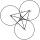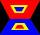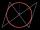# Triangle Problems

#### Number of problems found: 1272

• Isosceles right triangleCalculate the area of an isosceles right triangle whose perimeter is 252 cm.
• Widescreen monitorComputer businesses hit by a wave of widescreen monitors and televisions. Calculate the area of ​​the LCD monitor with a diagonal size 20 inches at ratio 4:3 and then 16:9 aspect ratio. Is buying widescreen monitors with the same diagonal more advantageou
• Glass mosaicHow many dm2 glass is nessesary to produc 97 slides of a regular 6-gon, whose side has length 21 cm? Assume that cutting glass waste is 10%.
• Triangle anglesThe angles α, β, γ in triangle ABC are in the ratio 6:2:6. Calculate size of angles.
• TangentsTo circle with a radius of 41 cm from the point R guided two tangents. The distance of both points of contact is 16 cm. Calculate the distance from point R and circle centre.
• RT - hypotenuse and altitudeRight triangle BTG has hypotenuse g=117 m and altitude to g is 54 m. How long are hypotenuse segments?
• Floating barrelBarrel (cylinder shape) floats on water, top of the barrel is 8 dm above water, and the width of surfaced barrel part is 23 dm. Barrel length is 24 dm. Calculate the volume of the barrel.
• CirclesThree circles of radius 95 cm 78 cm and 64 cm is mutually tangent. What is the perimeter of the triangle whose vertices are centers of the circles?
• Triangle ABCRight triangle ABC with right angle at the C, |BC|=18, |AB|=33. Calculate the height of the triangle hAB to the side AB.
• Rectangular trapezoidHow many inner right angles have a rectangular trapezoid?
• KitesBoys run kite on a cable of 68 meters long. What is the kite altitude, if the angle from the horizontal plane is 72°?Between cities A and B is route 13 km long of stúpanie average 7‰. Calculate the height difference of cities A and B.
• Angles in triangleThe triangle is ratio of the angles β:γ = 6:8. Angle α is 40° greater than β. What are the size of angles of the triangle?
• Circle chordWhat is the length x of the chord circle of diameter 115 m if the distance from the center circle is 11 m?
• Triangle SASCalculate the triangle area and perimeter, if the two sides are 51 cm and 110 cm long and angle them clamped is 130 °.
• Circle in rhombusIn the rhombus is an inscribed circle. Contact points of touch divide the sides to parts of length 19 cm and 6 cm. Calculate the circle area.
• Square diagonalCalculate the length of the square diagonal if the perimeter is 172 cm.
• Cube - anglesCalculate angle between the wall diagonal and cube base. Calculate the angle between the cube body diagonal and cube base.
• RhombusCalculate the perimeter and area of ​​a rhombus whose diagonals are 39 cm and 51 cm long.
• TrianglesEquilateral triangle with side 40 cm has the same perimeter as an isosceles triangle with arm of 45 cm. Calculate the base x of an isosceles triangle.

Do you have an exciting math question or word problem that you can't solve? Ask a question or post a math problem, and we can try to solve it.

We will send a solution to your e-mail address. Solved examples are also published here. Please enter the e-mail correctly and check whether you don't have a full mailbox.

Please do not submit problems from current active competitions such as Mathematical Olympiad, correspondence seminars etc...